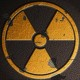# Looking at Clojure Koans

I worked on the Clojure koans.

The sixth file had this function:

Then one of the problems was like this;

I admit that I copied and pasted it.

I am having a hard time figuring this one out. If you call square, you would do it like this:

But in the answer, “square” is at the end. It seems like to get inline functions with higher-order functions you have to alternate between reading left-to-right and right-to-left. So I will try to parse it myself.

Here is the function:

((fn [f] (f 5)) square)

So let’s get started.

So first we have the inline function:

(fn [f] (f 5))

So we are declaring a function that takes an argument of 5. And so “square” is the argument. I guess that even though the name of the function is a string, that if the string matches a function name then Clojure will realize it’s a function.

So let’s see what might happen with this code runs (keep in mind I have not actually looked at the Clojure source code or tried to run any sort of debugger; I am not sure if Clojure has or needs a debugger).

Here is the function again:

((fn [f] (f 5)) square)

So let’s get started.

Let’s put the argument into the definition:

(fn [square] (f 5))

Now let’s put the argument into the function body:

(square 5)

Somehow this exercise is not as enlightening as I thought it would be. I don’t know if this sequence could be used by someone to figure out the koan.

To paraphrase Barbi: Clojure is hard.

## One Reply to “Looking at Clojure Koans”

1.Mark Johnson says:

I think working from the known solution and understanding it isn’t too hard. When you look at the body of the anonymous function, you see that the formal argument,f, is in the function position, the first position in the list.

I think the difficulty is in learning the vocabulary and terminology of FP. It is very different and foreign.